### Home > CALC > Chapter 8 > Lesson 8.1.4 > Problem8-42

8-42.
1. No calculator! Evaluate the integrals below. Homework Help ✎1.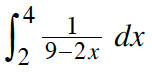2.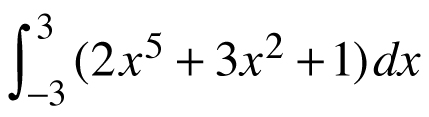3.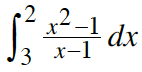4.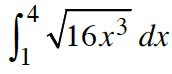5.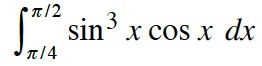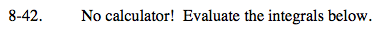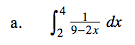Before integrating, make a choice:

$\text{You could factor out }-\frac{1}{2}.$

or You could let U = 9 − 2x.

$\int \frac{1}{x}dx=\text{ln}\left | x \right |+C$

Don't forget the absolute value!

$\text{ln}|a|-\text{ln}|b|=\text{ln}\left | \frac{a}{b} \right |$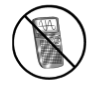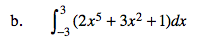Notice the symmetrical bounds.Is this function odd? If so, the area will be 0. If not, integrate.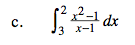Factor before integrating.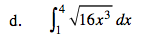$\text{Recall that }\sqrt{16x^{3}}=4x^{\frac{3}{2}}$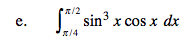U-substitution. Should U = sinx or U = cosx?